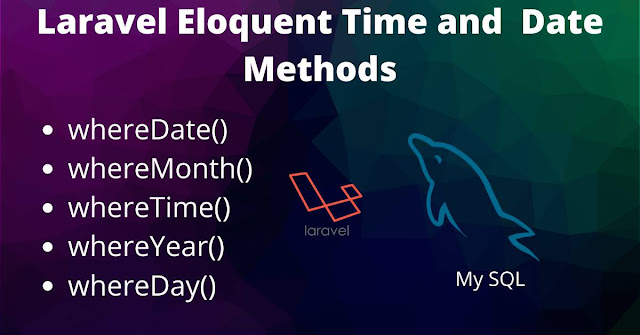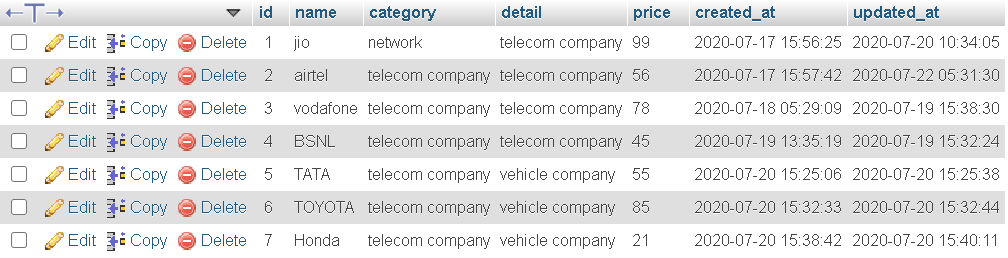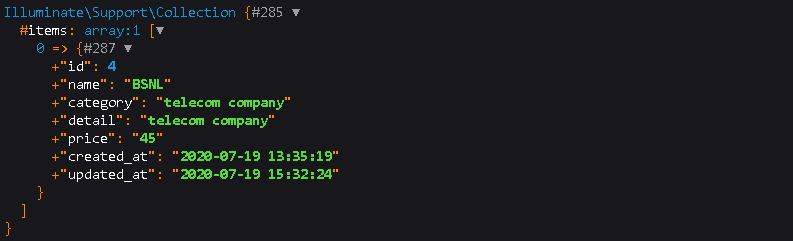# Laravel 7 Date Filtering Made Simple

0

## Laravel 7/6/5 whereDate | whereMonth | whereTime | whereYear | whereDay MethodsIn this article we will see how to use different Date and Time methods with where condition in Laravel . We will go through each methods with a proper example for better understanding .

As we know while inserting data or updating data in your database table Laravel automatically creates two fields that are ' created_at ' and ' updated_at ' . These two fields are very important while we need some data with the help of these Date and Time .

In this article we will cover these following Date and Time Methods with Examples .

#### whereDate() whereDay() whereMonth() whereYear() whereTime()

For understanding these methods we will take the example of following database table .### 1 - Laravel whereDate() :

whereDate() method basically takes  two parameter that is the column name and the value and returns the rows as an array which satisfy the condition .

### Syntax :

```\$data=DB::table('table_name')
->whereDate('column_name','vlaue')
->get();

dd(\$data);
```

### Example :

```\$data=DB::table('products')
->whereDate('created_at','2020-07-17')
->get();

dd(\$data);
```

### 2 - Laravel whereDay() :

whereDay() method takes two parameter that is column_name and value and and returns the rows that satisfies the condition or matches the value .

### Syntax :

```\$data=DB::table('table_name')
->whereDay('column_name','vlaue')
->get();

dd(\$data);
```

### Example :

```\$data=DB::table('products')
->whereDay('created_at','18')
->get();

dd(\$data);
```

### 3 - Laravel whereMonth() :

whereMonth() method takes two parameter that is column_name and value and and returns the rows that satisfies the condition or matches the value .

### Syntax :

```\$data=DB::table('table_name')
->whereMonth('column_name','vlaue')
->get();

dd(\$data);
```

### Example :

```\$data=DB::table('products')
->whereMonth('created_at','7')
->get();

dd(\$data);
```

### 4 - Laravel whereYear() :

whereYear() method takes two parameter that is column_name and value and and returns the rows that satisfies the condition or matches the value .

### Syntax :

```\$data=DB::table('table_name')
->whereYear('column_name','vlaue')
->get();

dd(\$data);
```

### Example :

```\$data=DB::table('products')
->whereYear('created_at','18')
->get();

dd(\$data);
```

### 5 - Laravel whereTime() :

whereTime() method takes two parameter that is column_name and value and and returns the rows that satisfies the condition or matches the value .

### Syntax :

```\$data=DB::table('table_name')
->whereTime('column_name','vlaue')
->get();

dd(\$data);
```

### Example :

```\$data=DB::table('products')
->whereTime('created_at','13:35:19')
->get();

dd(\$data);
```

### Output :These the 5 different types of Date-Time methods you can use in Laravel .Hope it helped you.